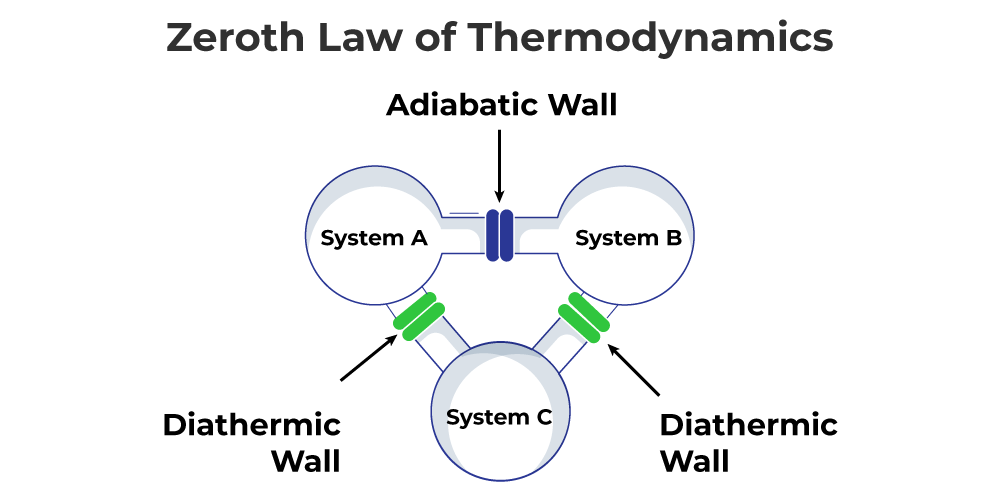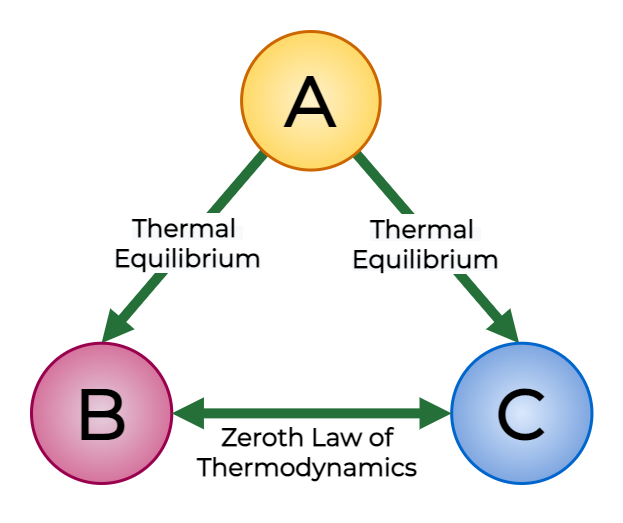GeeksforGeeks App
Open AppBrowser
Continue

## Related Articles

• CBSE Class 11 Physics Notes

# Zeroth Law of Thermodynamics

Zeroth Law of Thermodynamics states that when two bodies are in thermal equilibrium with another third body than the two bodies are also in thermal equilibrium with each other. Ralph H. Fowler developed this law in the 1930s, many years after the first, second, and third laws of thermodynamics had achieved widespread acceptance. Here Thermal Equilibrium implies that when two systems are in contact with a barrier permeable only to heat, so there will be no change or transfer of heat. Let’s learn more about Zeroth’s Law of Thermodynamics in this article!

## What is Zeroth Law of Thermodynamics?

Consider two systems A and B, separated by a wall that does not allow any exchange of energy between them. Such a wall is known as an insulating wall or adiabatic wall. The third system C is separated from systems A and B by a conducting or diathermic wall as shown in the figure.Since energy can be exchanged between systems A and C, both A and C are in thermal equilibrium. Similarly, energy can be exchanged between the systems B and C, so both B and C are also in thermal equilibrium. In other words, both systems A and B are in thermal equilibrium with the third system C separately. When the adiabatic wall between systems A and B is removed, no transfer of energy takes place between them. This shows that systems A and B are also in thermal equilibrium with each other. This observation leads to an important law known as Zeroth’s law of thermodynamics which is stated as follows:

Zeroth Law of Thermodynamics states that when two bodies are in thermal equilibrium with another third body then the two bodies are also in thermal equilibrium with each other.

According to Zeroth’s law of thermodynamics, if system A is in thermal equilibrium with system C, thenTemperature of System A = Temperature of System C

Similarly, if system B is in thermal equilibrium with system C, then

Temperature of System B =Temperature of System C

Now, from the above relation, we have

Temperature of System A = Temperature of System B

## Thermal Equilibrium

The zeroth law of thermodynamics recognizes that temperature is a valuable measurement since it predicts whether or not heat will flow between things. Regardless matter how the items interact, this is true. Heat can move between two things even if they are not physically interacting, as per the radiation method of heat transmission.

The zeroth law of thermodynamics says that no heat flow will occur if the systems are in thermal equilibrium.

Thermodynamics is distinguished from other studies by temperature. This trait is capable of distinguishing between hot and cold. When two or more bodies of different temperatures come into touch, they eventually reach a similar temperature and are said to be in thermal equilibrium.

## Zeroth Law of Thermodynamics Explanation

Zeroth Law of Thermodynamics states that heat energy flows between two bodies when they are kept in contact with each other.

For example, if we take three bodies P, Q, and R at different temperatures which are in contact with each other then the heat transfers among the three bodies till they reach thermal equilibrium, in this case, they reach the constant temperature.

Thermal equilibrium can also be achieved even when the temperature of the bodies is different. For example, if we take two bodies A and B, and contain them in adiabatic chambers (which do not allow heat to pass through them) then the, if they allow being in contact still there, is no heat transfer as adiabatic chambers do not allow heat to pass through it so they are considered to in thermal equilibrium.

The zeroth law of thermodynamics follows the translation relation between various bodies i.e. if we take three bodies A, B, and C then if A and B are in thermal equilibrium and B and C are in thermal equilibrium then A and c are also considered to be in thermal equilibrium.

## Application of Zeroth Law of Thermodynamics

The law is crucial for the mathematical formulation of thermodynamics, or, to put it another way, for expressing the mathematical definition of temperature. The most common use of this concept is to compare the temperatures of different things. If we want to accurately measure temperature, we’ll need a reference body and a characteristic of that body that changes with temperature. The change in that characteristic could be interpreted as a temperature change. The chosen feature is referred to as a thermodynamic property.

Nonetheless, Thermometers are the most prevalent application of the zeroth law of thermodynamics. Using a simple thermometer with mercury in a tube, we may witness the zeroth law in operation. Because the area of the tube remains constant as the temperature rises, the mercury expands. The height has grown as a result of this development. Now, the variation in the height of the mercury label indicates temperature changes and, in essence, aids us in measuring it.

Depending on their thermometric characteristic, several types of thermometers can be utilized. The following is a list of thermometers

• Constant pressure gas thermometer – Volume
• Constant volume gas thermometer – Pressure
• Electrical resistance thermometer – Resistance
• Mercury-in-glass thermometer – Length
• Thermocouple – Thermal e.m.f.

When you have two glasses of water, you have another illustration of the zeroth law of thermodynamics. One glass will be filled with hot water, while the other will be filled with cold water. If we leave them on the table for a few hours, they will achieve thermal equilibrium with the room’s temperature.

Related Articles

## FAQs on Zeroth Law of Thermodynamics

### Question 1: Define Zeroth Law of Thermodynamics.

According to the Zeroth Law of Thermodynamics, two systems that are in thermal equilibrium with a third system separately are also in thermal equilibrium with each other.

### Question 2: Who gave the Zeroth Law of Thermodynamics?

The credit for stating the zeroth law of Thermodynamics goes to British scientist Ralph H. Fowler.

### Question 3: What is the Significance of the Zeroth Law of Thermodynamics?

Zeroth Law of Thermodynamics provides a significant relationship which is very helpful for the mathematical formulation of thermodynamics. It mathematically transforms the relationship between systems’ thermal equilibrium into an equivalence relation, which can represent the equality of a particular quantity related to each system.

### Question 4: How does Zeroth Law lead to the concept of temperature?

Zeroth law tells that heat always flows from a hot body to a cold body till both bodies have an equal temperature. The degree of hotness or coldness is called the temperature and thus, the zeroth law of thermodynamics leads to the temperature.

### Question 5: Why is it called the “zeroth law” of thermodynamics?

This law of thermodynamics was explained after the other two laws of thermodynamics and should be named the third law of thermodynamics, but scientists discussed and concluded that this law is the most fundamental law of thermodynamics and should be named the first law but I and II law are already named whose instead of renaming both the laws scientist decided to named the newly formed law as the zeroth law and thus it is named the zeroth law.

### Question 6: What is the difference between Heat and Temperature?

Difference between Heat and Temperature are,

• Heat is a form of energy that is transferred between two bodies because if there is a temperature difference between them.
• Temperature is degree of hotness and coldness of the body and it may be defined as the thermal condition of a body that determines the direction of heat flow i.e. it determines whether the body will receive heat from another body or it will give heat to the same.